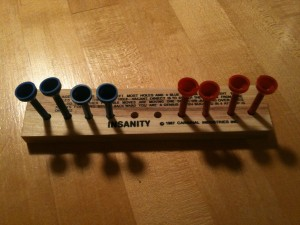Well, probably not the kind you’re expecting: Family drama, stress, gift anxiety, etc. No, I’m talking about “Insanity,” a game you probably haven’t heard of. It’s pretty simple. Ten holes on a piece of wood. Eight pegs; four red, four blue. Move all the pegs so that the colors are exactly switched. Pegs can only move in one direction. They can either move to an adjacent empty hole, or jump one peg of any color to an empty hole.

I picked it up and thought, gee, I could pretty quickly write a program to solve this. The game parameters were simple, yet it was a challenging enough problem that I couldn’t quickly pick it up and solve it. So I sat down with my laptop, fired up TextMate, and started hacking away. Python was the language of choice, mostly because I have a couple projects going on using it and needed the practice.My algorithm is about as dumb as they get: Choose a peg at random, then randomly do a jump or “slide” move, if possible. I figured there weren’t that many possible moves, so it wouldn’t take that long. A few minutes of tweaking, and the program would keep getting stuck because of some poor initial moves. A little more tweaking (mostly increasing the number of tries per game) and it finally found a solution. Success!

See the code below. There’s undoubtedly substantial room for improvement, but it does the job, usually solving the problem after 5-10 minutes. I was pretty happy about the number of mistakes I didn’t make, after getting familiar with the python interpreter. A fun diversion on a snowy Christmas day.

insanity.py

```1 2 3 4 5 6 7 8 9 10 11 12 13 14 15 16 17 18 19 20 21 22 23 24 25 26 27 28 29 30 31 32 33 34 35 36 37 38 39 40 41 42 43 44 45 46 47 48 49 50 51 52 53 54 55 56 57 58 59 60 61 62 63 64 65 66 67 68 69 70 71 72 73 74 75 76 77 78 79 80 81 82 83 84 85 86 87 88 89 90 91 92 93 94 95 96 97 98 99 100 101 102 103 104 105 106 107 108 109 110 111 112 113 114 115 116 117 import random   def play_game(): won = False while not won: game = Insanity() count = 0 while count < 10000: if game.game_over(): won = True print game.log print "You win!" game.move() count += 1 if game.moves > 25: print game.log   class Insanity: def __init__(self): self.holes = [] self.pegs = [] self.log = "" self.moves = 0 self.moved = False for i in range(10): h = Hole(i) if h.peg: self.pegs.append(h.peg) self.holes.append(h)   def move(self): self.moved = False peg_to_move = self.pegs[int(random.random() * 8)] r = random.random() if r > 0.5: if self.can_jump(peg_to_move): self.jump(peg_to_move) elif self.can_slide(peg_to_move): self.slide(peg_to_move) else: if self.can_slide(peg_to_move): self.slide(peg_to_move) elif self.can_jump(peg_to_move): self.jump(peg_to_move) if self.moved: self.log += self.print_board()   def jump(self, peg, distance=2): self.holes[peg.pos].peg = None new_pos = peg.pos + (distance * peg.direction()) self.holes[new_pos].peg = peg peg.pos = new_pos self.moved = True self.moves += 1   def slide(self, peg): self.jump(peg, 1)   def can_jump(self,peg): return peg.can_jump() and not self.holes[peg.pos + peg.direction()].is_empty() and self.holes[peg.pos + (2 * peg.direction())].is_empty()   def can_slide(self,peg): return not peg.at_end() and self.holes[peg.pos + peg.direction()].is_empty()   def print_board(self): stri = "" for hole in self.holes: stri += hole.__str__() return stri + "\n"   def game_over(self): for hole in self.holes[0:4]: if hole.is_empty() or not hole.peg.color == "red": return False for hole in self.holes[6:]: if hole.is_empty() or not hole.peg.color == "blue": return False return True   class Peg: def __init__(self, color, pos): self.color = color self.pos = pos   def at_end(self): return (self.color == "blue" and self.pos == 9) or (self.color == "red" and self.pos == 0)   def can_jump(self): return (self.color == "blue" and self.pos < 8) or (self.color == "red" and self.pos > 1)   def direction(self): return 1 if self.color == "blue" else -1   def p_color(self): return "B" if self.color == "blue" else "R"   def __str__(self): return "P(%i%s)" % (self.pos, self.p_color(),)   class Hole: def __init__(self, i): self.num = i if i < 4: self.peg = Peg("blue", i) elif i > 5: self.peg = Peg("red", i) else: self.peg = None   def is_empty(self): return self.peg == None   def __str__(self): p = "E" if self.peg: p = self.peg.p_color() return "%i%s " % (self.num, p,)```
One Response
1.